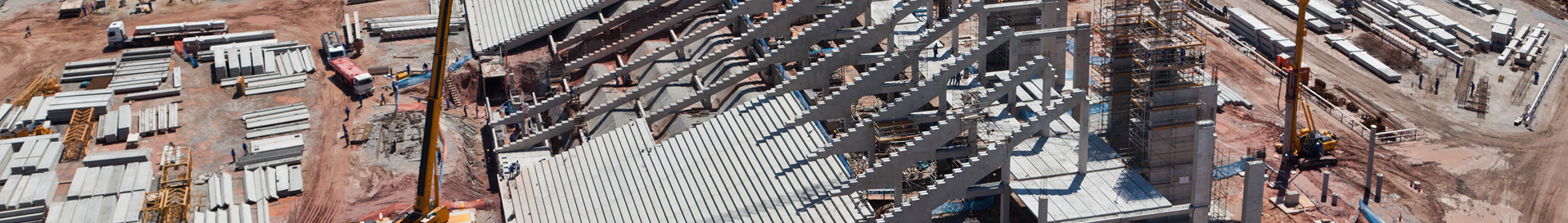•# Latest news

•# Structural Concrete, Vol. 10, no. 2, June 2009

## Design equation for predicting fire resistance of reinforced concrete columns

V.K.R. Kodur, Michigan State University, East Lansing, MI, USA
N. K. Raut, Michigan State University, East Lansing, MI, USA

An empirical equation for evaluating the fire resistance of reinforced concrete (RC) columns is presented. Data from a large set of experimental studies are analysed to study the influence of various parameters on the fire resistance of RC columns. The fire test data are utilised to develop a simplified equation for expressing the fire resistance of RC columns as a function of influencing parameters. The validity of the equation is established by comparing the predictions from the empirical equation with data obtained from fire resistance experiments and analytical studies. Predictions from the proposed equation are in good agreement with the test results and computer models, and provide better estimates of fire resistance than those predicted from current codes of practice. The proposed equation also incorporates parameters such as load eccentricity, which is not included in the current equations available in the literature. Furthermore, the proposed equation expresses the fire resistance in terms of conventional structural and material design parameters, and thus facilitates easy calculation of fire resistance.

Case postale 88
CH-1015 Lausanne
Switzerland

#### Contact

p : +41 21 693 27 47
f : +41 21 693 62 45
e : info@fib-international.org
w : www.fib-international.org